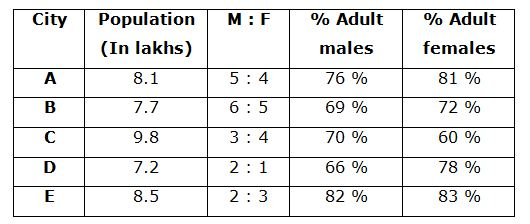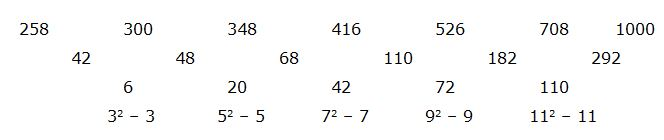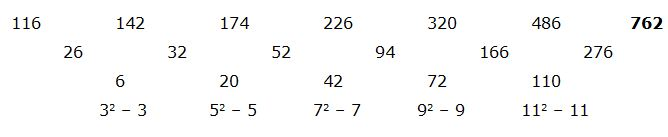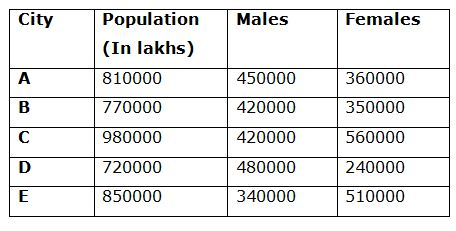# SBI PO Prelims Quantitative Aptitude Questions 2019 – Day 23

SBI PO 2019 Notification will be expected soon. It is one of the most expected recruitment among the banking aspirants. Every year the exam pattern for SBI PO has been changing. Depends upon the changing of exam pattern the questions are quite harder compare to the previous year. So the questions are in high level than the candidate’s assumption.

As per the latest trend, our IBPS Guide is providing the updated New Exam Pattern Quantitative Aptitude questions for SBI PO 2019 Day 23. Our Skilled experts were mounting the questions based on the aspirant’s needs. So candidates shall start your preparation and practice on daily basis with our SBI PO pattern quantitative aptitude questions 2019 day 23. Start your effective preparation from the right beginning to get success in upcoming SBI PO 2019.

[WpProQuiz 5449]

### Click Here for SBI PO Pre 2019 High-Quality Mocks Exactly on SBI Standard

Directions (1 – 5): The questions below are based on the given Series-I. The series-I satisfy a certain pattern, follow the same pattern in Series-II and answer the questions given below.

1)

5                 7                 25               131             925             8335

–                  ?                 –                  –                  –                  14005

a) 24

b) 35

c) 13

d) 42

e) 38

2)

258             300             348             416             526             708       1000

116             142             –                  –                  –                  –              ?

a) 762

b) 815

c) 927

d) 1033

e) 1128

3)

127             139             159             189             231             287        359

313             325             –                  –                  –                  –             ?

a) 638

b) 712

c) 596

d) 545

e) 820

4)

52               51               94               255             956             4655

?                 –                  –                  –                  –                  -25

a) 27

b) 13

c) 32

d) 40

e) 45

5)

36000        7200          720             48               2.4

–                  ?                 –                  –                  0.6

a) 3200

b) 2700

c) 1800

d) 3600

e) 1200

Directions (Q. 6 – 10): Study the following information carefully and answer the given questions:

The following table shows the population of different cities (In lakhs) and the ratio of males and females among them and the percentage of adult males and adult females.6) Find the difference between the total adult males in City C to that of total adult females in City A?

a) 3400

b) 2400

c) 6500

d) 4200

e) None of these

7) Find the ratio between the total number of males to that of total number of females of all the given cities together?

a) 178: 197

b) 151: 176

c) 256: 163

d) 211: 202

e) None of these

8) Total number of minor males in City A and B together is approximately what percentage of total number of minor females in City D and E together?

a) 155 %

b) 185 %

c) 200 %

d) 170 %

e) 215 %

9) Total number of adult females in City B and C together is approximately what percentage more/less than the total number of adult males in City A and E together?

a) 5 % less

b) 15 % more

c) 15 % less

d) 30 % more

e) 5 % more

10) Find the average number of adult females in all the given cities together?

a) 324840

b) 259360

c) 389280

d) 298020

e) None of these

Directions (1-5):

Series I pattern:

5*1 + 2 = 7

7*3 + 4 = 25

25*5 + 6 = 131

131*7 + 8 = 925

925*9 + 10 = 8335

Series II pattern:

(14005 – 10)/9 = 1555

(1555 – 8)/7 = 221

(221 – 6)/5 = 43

(43 – 4)/3 = 13

(13 – 2)/1 = 11

Series I pattern:The difference of difference is, 32 – 3, 52 – 5, 72 – 7, 92 – 9, 112 – 11,…

(or)

The difference of difference is, (2*3), (4*5), (6*7), (8*9), (10*11)

Series II pattern:The difference of difference is, 32 – 3, 52 – 5, 72 – 7, 92 – 9, 112 – 11,…

(or)

The difference of difference is, (2*3), (4*5), (6*7), (8*9), (10*11)

Series I pattern:

127 + (3*4) = 139

139 + (4*5) = 159

159 + (5*6) = 189

189 + (6*7) = 231

231 + (7*8) = 287

287 + (8*9) = 359

The pattern is, +(3*4), +(4*5), +(5*6), +(6*7), +(7*8), +(8*9),…

(Or)

The difference of difference is, 8, 10, 12, 14, 16,….

Series II pattern:

313 + (3*4) = 325

325 + (4*5) = 345

345 + (5*6) = 375

375 + (6*7) = 417

471 + (7*8) = 473

473 + (8*9) = 545

Series I pattern:

52*1 – 13 = 51

51*2 – 23 = 94

94*3 – 33 = 255

255*4 – 43 = 956

956*5 – 53 = 4655

Series II pattern:

(-25 + 125)/5 = 20

(20 + 64)/4 = 21

(21 + 27)/3 = 16

(16 + 8)/2 = 12

(12 + 1)/1 = 13

Series I pattern:

36000 ÷ 5 = 7200

7200 ÷ 10 = 720

720 ÷ 15 = 48

48 ÷ 20 = 2.4

Series II pattern:

0.6*20 = 12

12*15 = 180

180*10 = 1800

1800*5 = 9000

(Or)

2.4/4 = 0.6, So, 7200/4 = 1800

Directions (6-10):

The total number of adult males in City C

= > 980000*(3/7)*(70/100) = 294000

The total number of adult females in City A

= > 810000*(4/9)*(81/100) = 291600

Required difference = 294000 – 291600 = 2400The total number of males of all the given cities together

= > (4.5 + 4.2 + 4.2 + 4.8 + 3.4) lakhs

= > 21.1 lakhs

= > 2110000

The total number of females of all the given cities together

= > (3.6 + 3.5 + 5.6 + 2.4 + 5.1) lakhs

= > 20.2 lakhs

= > 2020000

Required ratio = 2110000: 2020000 = 211: 202

Total number of minor males in City A and B together

= > 8.1*(5/9)*(24/100) + 7.7*(6/11)*(31/100)

= > (1.08 + 1.302) lakhs

= > 2.382 lakhs

= > 238200

Total number of minor females in City D and E together

= > 7.2*(1/3)*(22/100) + 8.5*(3/5)*(17/100)

= > (0.528 + 0.867) lakhs

= > 1.395 lakhs

= > 139500

Required % = (238200/139500)*100 = 170.75 % = 170 %

Total number of adult females in City B and C together

= > 7.7*(5/11)*((72/100) + 9.8 *(4/7)*(60/100)

= > (2.52 + 3.36) lakhs

= > 5.88 lakhs

Total number of adult males in City A and E together

= > 8.1*(5/9)*(76/100) + 8.5*(2/5)*(82/100)

= > (3.42 + 2.788) lakhs

= > 6.208 lakhs

Required % = [(6.208 – 5.88)/6.208]*100

= > 5.28 % = 5 % less

Total number of adult females in all the given cities

= > 8.1*(4/9)*(81/100) + 7.7*(5/11)*(72/100) + 9.8*(4/7)*(60/100) + 7.2*(1/3)*(78/100) + 8.5*(3/5)*(83/100)

= > (2.916 + 2.52 + 3.36 + 1.872 + 4.233) lakhs

= > 14.901 lakhs

= > 1490100

Required average = 1490100/5 = 298020